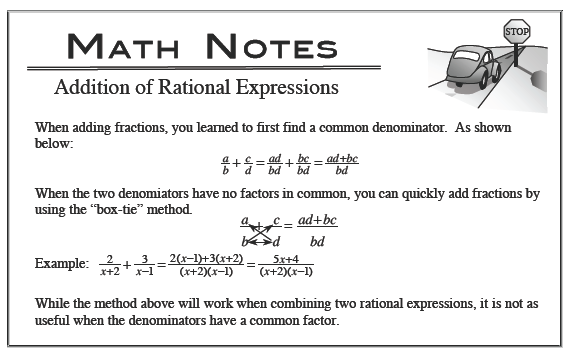### Home > PC > Chapter 2 > Lesson 2.3.1 > Problem2-77

2-77.
1. Find the following sums. Homework Help ✎

1.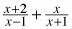2.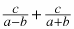3.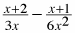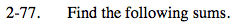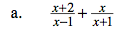$\frac{(x+2)(x+1)}{(x-1)(x+1)}+\frac{(x)(x-1)}{(x+1)(x-1)}$

$\frac{(x^2+x+2x+2)+(x^2-x)}{x^2+x-x-1}$

$\frac{2x^2+2x+2}{x^2-1}$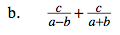Review the Math Notes box and follow the example.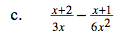Review the Math Notes box and follow the example.